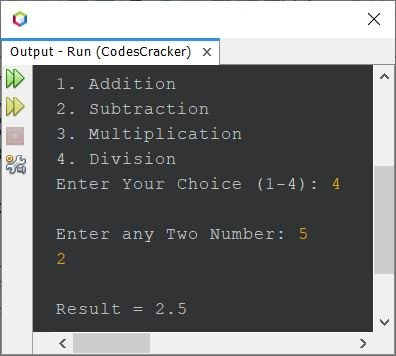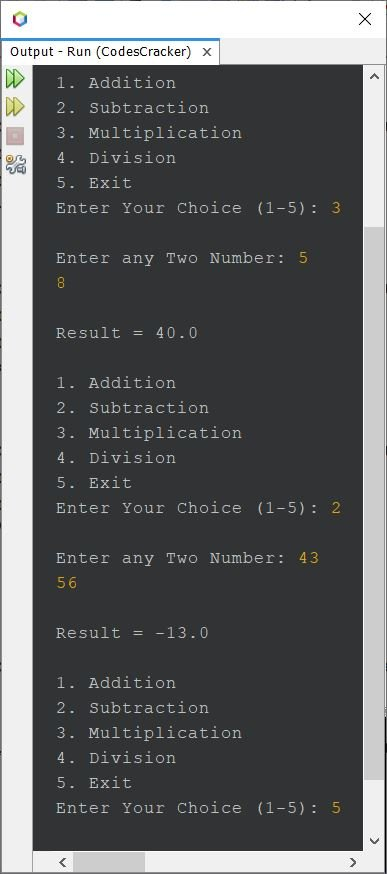# Simple Calculator Program in Java

• Calculator program in Java using if else
• Calculator program in Java using switch case - with infinite loop
• Calculator program in Java using functions and switch case

## Calculator Program in Java using if else

The question is, write a simple calculator program in Java that performs four basic arithmetic operations. The program given below is its answer:

```import java.util.Scanner;

public class CodesCracker
{
public static void main(String[] args)
{
float a, b, res;
int choice;
Scanner scan = new Scanner(System.in);

System.out.println("2. Subtraction");
System.out.println("3. Multiplication");
System.out.println("4. Division");
choice = scan.nextInt();

if(choice>=1 && choice<=4)
{
System.out.print("\nEnter any Two Number: ");
a = scan.nextFloat();
b = scan.nextFloat();

if(choice==1)
res = a+b;
else if(choice==2)
res = a-b;
else if(choice==3)
res = a*b;
else
res = a/b;

System.out.println("\nResult = " +res);
}
else
System.out.println("\nInvalid Choice!");
}
}```

The snapshot given below shows the sample run of above program, with user input 4 as choice, 5 and 2 as two numbers, where 5 as first and 2 as second number:## Calculator Program in Java using switch

Here is another calculator program in Java created using switch case, instead of if...else. This program also continue its execution, until user enters 5 as choice to exit or stop the execution.

```import java.util.Scanner;

public class CodesCracker
{
public static void main(String[] args)
{
float a, b, res=0;
int choice;
Scanner scan = new Scanner(System.in);

while(true)
{
System.out.println("2. Subtraction");
System.out.println("3. Multiplication");
System.out.println("4. Division");
System.out.println("5. Exit");
choice = scan.nextInt();

switch(choice)
{
case 1:
System.out.print("\nEnter any Two Number: ");
a = scan.nextFloat();
b = scan.nextFloat();
res = a+b;
break;
case 2:
System.out.print("\nEnter any Two Number: ");
a = scan.nextFloat();
b = scan.nextFloat();
res = a-b;
break;
case 3:
System.out.print("\nEnter any Two Number: ");
a = scan.nextFloat();
b = scan.nextFloat();
res = a*b;
break;
case 4:
System.out.print("\nEnter any Two Number: ");
a = scan.nextFloat();
b = scan.nextFloat();
res = a/b;
break;
case 5:
return;
default:
System.out.println("\nInvalid choice!");
break;
}
System.out.println("\nResult = " +res+ "\n");
}
}
}```

Here is its sample run with some user inputs to perform the calculation:## Calculator Program in Java using Functions and switch

This program is created using user-defined functions. This program works exactly same as of previous program.

```import java.util.Scanner;

public class CodesCracker
{
public static void main(String[] args)
{
float a, b, res=0;
int choice;
Scanner scan = new Scanner(System.in);

while(true)
{
init();
choice = scan.nextInt();

switch(choice)
{
case 1:
msg();
a = scan.nextFloat();
b = scan.nextFloat();
break;
case 2:
msg();
a = scan.nextFloat();
b = scan.nextFloat();
res = sub(a, b);
break;
case 3:
msg();
a = scan.nextFloat();
b = scan.nextFloat();
res = mul(a, b);
break;
case 4:
msg();
a = scan.nextFloat();
b = scan.nextFloat();
res = div(a, b);
break;
case 5:
return;
default:
System.out.println("\nInvalid choice!");
break;
}
System.out.println("\nResult = " +res+ "\n");
}
}
public static void init()
{
System.out.println("2. Subtraction");
System.out.println("3. Multiplication");
System.out.println("4. Division");
System.out.println("5. Exit");
}
public static void msg()
{
System.out.print("\nEnter any Two Number: ");
}
public static float add(float x, float y)
{
return x+y;
}
public static float sub(float x, float y)
{
return x-y;
}
public static float mul(float x, float y)
{
return x*y;
}
public static float div(float x, float y)
{
return x/y;
}
}```

This program produces same output as of previous program.

#### Same Program in Other Languages

Java Online Test

« Previous Program Next Program »